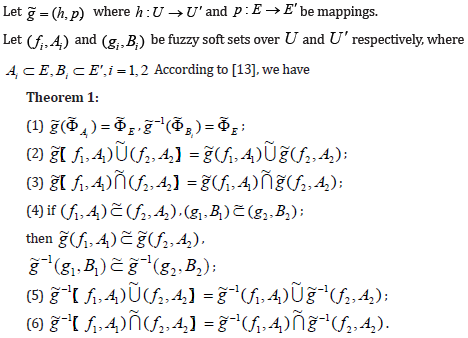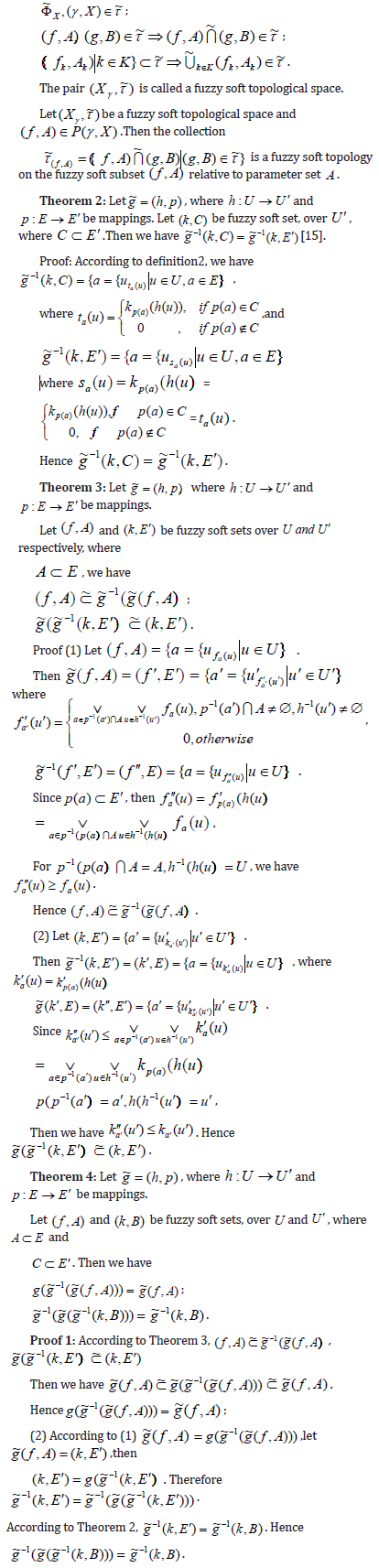• Submissions# Mappings on Fuzzy Soft Topological Spaces

Jingshui Ping*

School of Finance and Mathematics, China

*Corresponding author: Jingshui Ping, School of Finance and mathematics, Huainan, China

Submission: September 20, 2019; Published: September 30, 2019Volume2 Issue3
September, 2019

#### Abstract

This paper had been popularized the operational property of fuzzy soft mapping on the basis of fuzzy soft mapping. We had set up a kind of soft mapping and fuzzy soft continuous on fuzzy soft topology and discussed the application of fuzzy soft mapping and its composite properties on the construction of fuzzy soft topology.

Keywords: Fuzzy soft set; Fuzzy soft mapping; Fuzzy soft topology; Fuzzy soft continuous

#### Introduction

Molodtsov put forward the soft set theory in order to handling uncertain problems in 1999. This theory had overcome a main shortcoming of mathematical methods; that was lacking parameter tool. The soft set theory has been successfully applied in several fields such as decision analysis, pattern recognition and data collection. Maji  perfected the theoretical system of soft set and studied the fuzzy soft set theory. Paper included the research of the algebraic structure of soft set; and Hazra  had set up the soft topology construction. In Kharal & Ahmad  began to study fuzzy soft mapping. In 2011, Bekir T & Burc K  set up a fuzzy soft topology structure. In 2012, Abdülkadir  studied some properties of soft mapping on soft topology. Based on all these mentioned above, we research on fuzzy soft mapping on fuzzy soft topology and its application in fuzzy soft topology structure .

### Definition 1

 Let A ⊂ E and F(U)be the set of all fuzzy sets in U. Then the pair ( f , A) is called a fuzzy soft set over U , where f : A→ F(U) is a function. A fuzzy soft set ( f , A) can be viewed  (f,A) = {a = {ufa(u)|u∈ U} [8-12].

### Definition 2

13] Let g~ = (h, p) , where h :U →U′ and p : E → E′ be mappings. Let ( f , A) and (k,C) be fuzzy soft sets, over U and U′ , where A ⊂ E andC ⊂ E′

A fuzzy soft image of a fuzzy soft set ( f , A) under mapping g~ is defined as follows:A fuzzy soft inverse image of a fuzzy soft set (k,C) under mapping g is defined asRemark 1: The definition mentioned above, actually, is a kind of improvement of 3.2 definition in document .### Definition 3

 Let (γ , X ) be an element of F(U;E) , P(γ , X ) be the set of all fuzzy soft subsets of ) , ( X γ and τ ~ be a subfamily of P(γ , X ) .Then τ ~ is called fuzzy soft topology on (γ , X ) if the following conditions are satisfied:### Definition 4#### Conclusion

Fuzzy soft mapping is an extremely significant part in studying the spacious property of fuzzy soft topology. This paper popularizes fuzzy soft mapping as well as the operational properties of its inverse and composite operation. We set up the fuzzy soft continuous on fuzzy soft topology and study the application of fuzzy soft mapping’s various properties in the construction of fuzzy soft topology. As for the question that how to establish fuzzy soft quotient topology space by fuzzy soft mapping, detailed information will be given in author’s another paper.

﻿

We at Crimson Publishing are a group of people with a combined passion for science and research, who wants to bring to the world a unified platform where all scientific know-how is available read more...